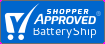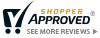Save 5% Extra Online. Use code: SAV81MenuSave 5% Extra Online. Use code: SAV81More Battery Answers

How Many Cells Are In A Battery

Determining how many cells are in a battery depends on the chemistry being used and how the battery is built. Most lithium based batteries are built with the cells in series and parallel. For example in a lithium based battery to determine the total number of cells in any battery you need to divide the nominal voltage by the battery's voltage. Next you need to divide the nominal capacity by the battery's capacity. Finally multiply the product of those two divisions and you will be able to determine the number of cells.

In a NICD or NIMH battery for example the cells are built in series. So you would need to divide the nominal voltage (typically 1.2V) by the total voltage and then the resulting number would be the quantity of the cells.